## Example Questions

1 2 3 4 5 6 8 Next →

### Example Question #71 : Probability

Harold is going to flip a coin seven times. What is the probability that he'll get a head three times and a tails four times?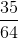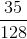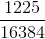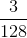Explanation:

This problem is dealing with the repeated trials for an event that only has two potential outcomes. This scenario describes a binomial distribution.

Fortrials, each of which has a probabilityof a 'successful' outcome, the probability of exactlysuccesses is given by the function: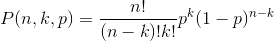Note that if Harold flips a coin and gets head exactly three times, he'll have to get four tails, and vice-versa. Calculate the probability of one and you get the probability of the other; there is no need to calculate both. The probability of getting heads is one half. For the three heads: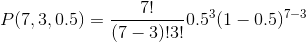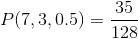### Example Question #72 : Probability

Jenn is flipping a coin ten times. What is the probability that she'll get heads at least once?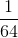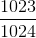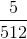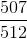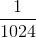Explanation:

This problem is dealing with the repeated trials for an event that only has two potential outcomes. This scenario describes a binomial distribution.

Fortrials, each of which has a probabilityof a 'successful' outcome, the probability of exactlysuccesses is given by the function:Now, when there is language such as 'at least' or 'no more than', a cumulative probability can be assumed. I.e. we sum up the probabilities of each event that satisfies the condition. For example, if we're asked for the probability of rolling a three on a die at least two times out of four, we'd sum up the probability of rolling  a three twice, a three thrice, and a three four times. If we're asked for the probability rolling a three no more than once out of four times, we'd sum up the probabilities of rolling a three once, and of rolling a three zero times.

Now, to calculate the probability of flipping a head at least once out of ten times, it would be time consuming to calculate the probability of once, twice, thrice, etc.

Realize, rather, that flipping the coin heads at least once is the complement to flipping it zero times. That is to say it is one minus the probability of flipping it zero times.

The probability of flipping heads is one half.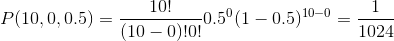So the complement, the probability of one or more heads, is### Example Question #73 : Probability

Able-bodied Abel is rolling a twenty-sided die to the best of his ability to defeat an orc that isn't quite sure how it got involved in this contest in the first place. Abel is rolling the die three times, and needs to roll a sixteen or greater at least twice in order to win. What is the probability of Abel winning?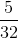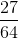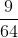Explanation:

This problem is dealing with the repeated trials for an event that only has two potential outcomes. This scenario describes a binomial distribution.

Fortrials, each of which has a probabilityof a 'successful' outcome, the probability of exactlysuccesses is given by the function:Now, when there is language such as 'at least' or 'no more than', a cumulative probability can be assumed. I.e. we sum up the probabilities of each event that satisfies the condition. For example, if we're asked for the probability of rolling a three on a die at least two times out of four, we'd sum up the probability of rolling  a three twice, a three thrice, and a three four times. If we're asked for the probability rolling a three no more than once out of four times, we'd sum up the probabilities of rolling a three once, and of rolling a three zero times.

The probability of rolling a sixteen or greater is. This can be seen by dividing the number of events that satisfy this by the number of total possible events: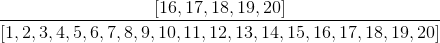Now, to get this sixteen or greater at least twice out of three rolls: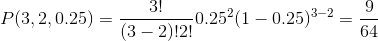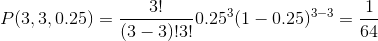The probability of Abel winning is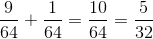Looks like the odds favor the orc.

### Example Question #74 : Probability

Aaron, Gary, Craig, and Boone are sitting down in a row of four chairs. What is the probability that Aaron and Gary will be seated beside each other?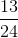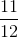Explanation:

Consider first all of the possible ways the men may be arranged, which is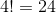Now, consider all of the ways that Aaron and Gary could be seated beside each other; it may be easier to visualize by drawing it out:

1. A G _ _
2. G A _ _
3. _ A G _
4. _ G A _
5. _ _ A G
6. _ _ G A

As seen, there are six possibilities.

Finally, for each of these cases, Craige and Boone could be seated in one of two ways.

So the probability that Aaron and Gary will be seated beside each other is: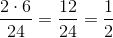### Example Question #75 : Probability

How many different seven-digit integers exist for which there are no repeated digits?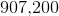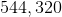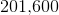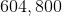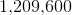Explanation:

To solve this problem, employ the fundamentals of combinatorics. For the first digit of the number, you might think that there are 10 options (the digits 0-9), but remember that no seven-digit integer would start with 0 (e.g. you don't represent one-hundred thousand as 0,100,000). So there are 9 choices available for the first digit.

For the second digit, there are also 9 choices: 0 is now a possibility, but you cannot repeat the original digit.

For each remaining spot, you lose one option because you cannot match the unique digits you've already chosen.  For the third digit you have two of the ten options unavailable because you're chosen two already; for the fourth digit you cannot match the previous three, and so on.  So the calculation becomes:

9 * 9 * 8 * 7 * 6 * 5 * 4 = 544,320.

### Example Question #76 : Probability

A 20-sided die is rolled 3 times in a row. What is the probability of rolling a 6 or greater at least twice?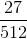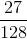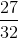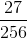Explanation:

This problem is dealing with binomial probabilities. For a probability of success,, andtrials, the probability ofsuccesses is: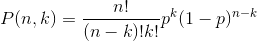For this problem there are three trials, and we're interested in at least two successes. Note that this probability equation gives the probability of getting EXACTLY k succeeses, so we must consider getting three successes at well.

The probability of rolling at least a six on a twenty-sided die is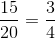Therefore, the probability of rolling this at least twice out of three trials is: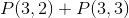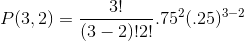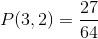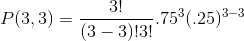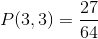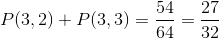1 2 3 4 5 6 8 Next →

Tired of practice problems?

Try live online GRE prep today.